• 马尔可夫过程 与 隐马尔科夫模型
千次阅读
2022-03-07 23:14:17

# 为什么是马尔可夫过程？

马尔科夫过程(Markov process)是一类随机过程。

在已知目前状态(现在)的条件下，它未来的演变(将来)不依赖于它以往的演变(过去)。主要研究一个系统的状况及其转移的理论。它是通过对不同状态的初始概率以及状态之间的转移概率的研究，来确定状态的变化趋势，从而达到对预测未来的目的。

# 概念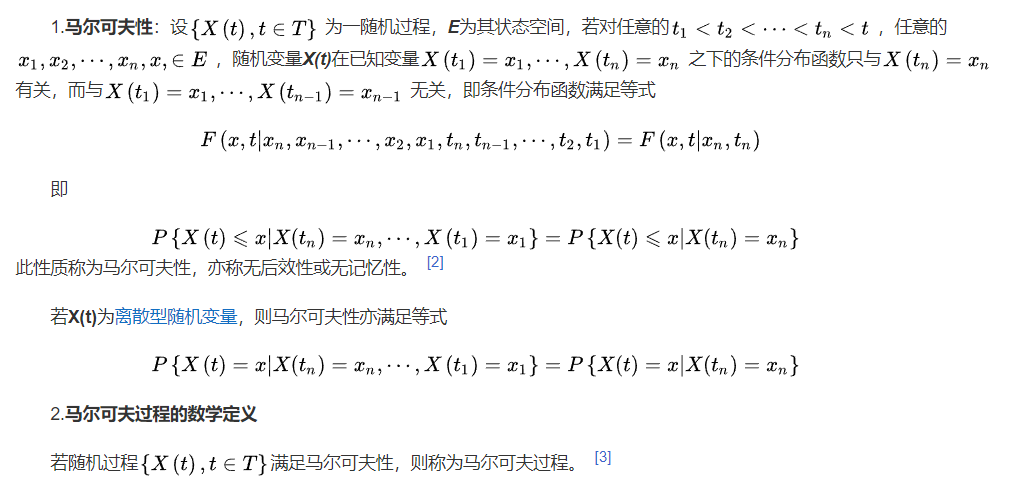• 液体中的微粒子运动
• 传染病的传染人数
• 车站的候车人数

# 两个基本特性

## 1. 无后效性

是指如果在某个阶段上过程的状态已知，则从此阶段以后过程的发展变化仅与此阶段的状态有关，而与过程在此阶段以前的阶段所经历过的状态无关。

我的一句话总结：未来的结果，不看过去，就看现在。

## 2. 便利性

不管事物出现在什么状态，较长一段时间内，马尔可夫过程逐渐趋于稳定，与初始状态无关。

# 马尔科夫链Markov chain

马尔科夫链(Markov chain) 是指具有马尔科夫性质的离散事件随机过程，即时间和状态参数都是离散的马尔科夫过程，是最简单的马尔科夫过程。

# 隐马尔科夫模型（重点）

隐马尔可夫模型(Hidden Markov Model, HMM)作为一种统计分析模型，创立于20世纪70年代。

一句话总结：隐马尔可夫模型，是统计学的，主要做统计分析的。

隐马尔可夫模型(Hidden Markov Model, HMM)是结构最简单的动态贝叶斯网这是一种著名的有向图模型，主要用于时序数据建模(语音识别、自然语言处理等）。

贝叶斯与朴素贝叶斯都是对隐马尔可夫模型做的铺垫。那两个就属于是“抛砖”了！
有向图：有明确的开始和结束，有方向
无向图：没有明确的开始和结束。
任何一个时序序列里面，有开始和结束时间。一定是有向图了！

隐马尔可夫模型是马尔可夫链的一种，它的状态不能直接观察到，但能通过观测向量序列观察到，每个观测向量都是通过某些概率密度分布表现为各种状态,每一个观测向量是由一个具有相应概率密度分布的状态序列产生。

马尔可夫链，就是描述一种状态的序列，每一个状态值，取决于前面的有限个状态

## 隐马尔科夫模型 模型图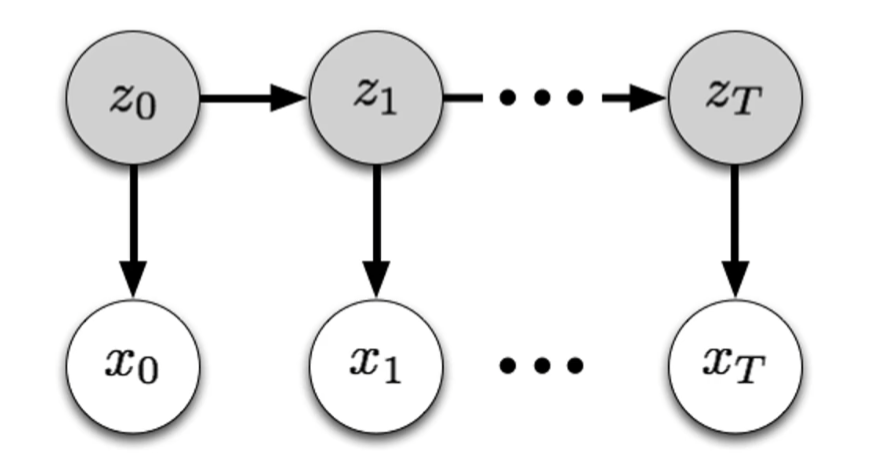箭头：表示了变量之间的依赖关系，也就是任意一个时刻，观测到变量的取值，仅依赖于该时刻的状态变量。
例如：x1z1来确定的，与其他变量的取值无关。
同时在T时刻，状态 zT 时刻，仅依赖于 T-1 时刻的状态。

说白了这仅是一个链式结构，当前时刻的状态，仅取决于前一个时刻，和其他时刻无关。和过往的，以前的，是没有任何关系的，不依赖的。

## 隐马尔科夫模型 的构成

隐马尔可夫模型由五个要素组成，其中两个状态集合(N、M)，三个概率矩阵(A、B、π)：

1. N，表示模型中的状态数，状态之间可以相互转移。

上图的 x0,x1,x2……xT

1. M，表示每个状态不同的观察符号，即输出字符的个数。

理解为 输出的个数

1. A，状态转移概率分布。

模型在各个状态之间转换的概率。例如：一个状态“好”，下一时刻状态“好”的概率是多少

1. B，观察符号在各个状态下的概率分布。

输出的观测概率值。模型根据当前状态所得出各个观测值的概率

1. π，表示初始状态分布。

初始时候，模型的各个状态都出现，出现的概率是多少

输入: HMMs的五元组(N, M，A, B, π)。
输出:–个观察符号的序列，这个序列的每个元素都是M中的元素。

输出都是 M，表示每个状态不同的观察符号。
根据概率求出一个输出值，把输出的内容会组成一个集合。输出的集合可以定位出M，每一个符号都是M种类的元素。

## 应用场景

• 中文分词：一句话中需要填空，“今天早上上语文课，我需要带一本（）书”，A.英语 B.数学 C.语文，通过这个状态，可以一个值一个值的概率预测，找到可能是“语文书”
• 机器翻译
• 语音识别
• 通信中的译码

隐马尔科夫模型 是传统的机器学习模型，并不属于深度学习模型，但深度学习模型很大程度上依赖于机器学习模型。
隐马尔科夫模型 也是非常常用的模型。

# 用一个小故事理解 隐马尔科夫模型

附：下面转引网络文章《爱情的隐式马尔可夫模型(Love in the Hidden Markov Model)》片断：

首先感谢原英文作者Tom Yeh的精彩描述，生动地讲述了HMM模型的原理，在此我斗胆用我自己的语言用中文修改描述一次。

男生和女生分别是来自不同星球的科学事实已经众所周知的了。男生们总是认为，女生们都是谜一样的生物，他们的情感状态浮动似乎是以秒单位在变化的，难以理解，更勿论预测了! 而女生们觉得男生都是没有感觉动物，完全不能理解什么叫感受-尽管已经告诉他们N次了!这种男女之间的根本差别，导致了他们之间的感情关系是受一种超级无敌复杂的系统所支配的。

不过，我们可以用一个叫隐式马尔可夫(Hidden Markov Model)的数学模型来分析这个系统。

小明，作为一名计算机科学家，决定要系统地去分析他女朋友的情感不确定性，挖掘出里面的规律!于是乎，小明仔细地记录了半年来他女朋友小丽每天的喜怒哀乐变化状态，并作了一张图表来表示小丽的历史情感变化。小明想知道，有了这些数据，他能否从中得出知道，如果小丽某天的情感状态是高兴，那么第二天她更多的是保持好心情呢，还是更多地变得悲伤了，如此等等……

数据胜于雄辩。小明从这半年的数据里面发现，当小丽高兴的时候，3/4的情况下第二天她仍然保持着好心情，只有1/4的情况小丽第二天心情会改变，比如变得气愤，悲伤等等。小明继续分析其他各种情感状态变化情况，比如从高兴到悲伤，悲伤到气愤，高兴到气愤等所有的可能组合。很快小明就得到所有的组合变化数据，从中得知对于任意小丽的某天情感状态下，下一个最有可能的情感状态。

这个过程，同学们，就是有名的 “马尔可夫过程” (Markov process)

不过需要注意的是，马尔可夫过程有一些假设的前提。在我们的例子里面，预测下一天小丽的心情，我们只依赖当天小丽的心情，而没有去考虑更先前她的心情。很明显这种假设下的模型是远不够精确的。很多时候，随着日子一天一天的过去，女生一般会变得越来越体谅。经常女生生气了几天后，气就会慢慢消了. 比方说如果小丽已经生气了3天了，那么她第二天变得高兴起来的可能性，在多数情况下，要比她只生气了一天而第二天变得高兴的可能性要高。马尔可夫过程并没有考虑这个，用行话讲，就是马尔可夫模型忽略远距离历史效应 ( long range dependency)。

有些时候，我们无法直接观测一个事物的状态。比方说，有些女生是很能隐瞒自己的情感而不流露出来的！她们可能天天面带微笑但不代表他们就天天高兴。因此我们必须要有窍门，去依赖某些我们能够直接观察到的东西。

话说回来，我们的主人公小明，自从被小丽发现他这种近乎变态的科学分析行为后，变得非常善于隐藏自己的心情，导致某天小明错误估计了小丽的心情！在误以为那天小丽会心情好的情况下，小明告诉小丽自己不小心摔坏了她心爱的iPod…，小明没想到其实那天小丽正因为前一天错过了商场名牌打折扣的活动而异常气愤。一场血雨腥风过后，两个人最终分手了。

不过，小明凭着自身的英俊高大潇洒，很快又交上了另外一个女朋友，小玲。鉴于小明意识到，女生表面的情感流露非常不可靠，小明决定要另寻他径，继续预测女朋友的心情！(作为一个科学家，小明的确有着不怕碰壁的精神)小明每个月都帮小玲付信用卡的费用(真不明白，有这样的男朋友，小玲有什么理由不高兴啊)，因此小明每天都可以通过Online banking知道小玲每天都买了什么东西。小明突然灵机一动: “没准我能通过观测她的购物规律，推导预测出小玲的心情！”

听起来有点匪夷所思，不过这个过程，的的确确是可以使用叫作隐式马尔可夫的数学模型来表示并分析的。

由于我们需要预测的变量 - 心情状态是无法直接观测的，是隐藏 (Hidden) 起来的，因此这种模型才叫隐式马尔可夫模型。

隐马尔可夫模型在计算机语音识别等领域取得了惊人的成功。据称：“到目前为止，HMM(隐马尔可夫模型)一直被认为是实现快速精确的语音系统的最成功的方法。”自然语言处理 人工智能 机器学习 算法
更多相关内容
• 机器学习中的隐马尔科夫模型的Python实现，包括参考资料链接等
• 使用此程序可用于模式识别中对数据信号的分类和预测
• HMM隐马尔科夫代码及其说明，很详细，初学者必备，可用来解决一系列问题
•matlab
• 基于隐马尔科夫模型的语音合成技术研究。随着目前语音合成效果的逐步改善，用户对语音合成系统提出了更高的要 求，尤其是多样化语音合成方面的需求。在这种背景下，一种能够在短时间内通 过自动训练的方式进行合成...语音合成
• 一份完全按照李航<<统计学习方法>>介绍的HMM代码，供大家参考，具体内容如下 #coding=utf8 ''''' Created on 2017-8-5 里面的代码许多地方可以精简，但为了百分百还原公式，就没有精简了。...
• 实现隐马尔科夫模型 包含前向后项算法、viterbi、baumWelch参数学习 c语言实现
• 隐马尔科夫模型基础
1. ​引言

隐马尔可夫模型(Hidden Markov model, HMM)是用于序列标注的概率图模型，描述一个隐藏的马尔科夫链生成不可观测的状态序列，再由每个状态生成一个观测而产生一个观测序列的过程，是一个生成模型。隐马尔可夫模型在自然语言处理、语音识别、模式识别等领域都应用广泛。在自然语言处理中，基于字标注的分词、词性标注、句法分析、命名实体识别等领域都可以应用隐马尔可夫模型。

虽然现在深度学习大行其道，HMM（在训练数据充足的情况下）也不如条件随机场（Conditional Random Field，CRF）强大，但是HMM依然是经典的统计分析模型，HMM包含的一些基本原理和概念，是学习其他算法的基础，比如随机采样中的马尔可夫-蒙特卡洛方法(Markov Chain Monte Carlo)用马尔科夫链产生样本序列，CRF中的随机场即马尔可夫随机场。因此，接下去我们简单的学习一下隐马尔科夫模型。

2.  隐马尔科夫模型的框架

隐马尔可夫模型的基础内容其实非常简单，总结起来只需要记住“1、2、3”，即1个元组，2个假设，3个问题。

2.1 一个元组

1个元组就是隐马尔可夫模型的参数元组，即组成隐马尔科夫模型的要素。一般来说是一个三元组  或者一个五元组  。五元组比三元组多了一个可能的状态集合Q和可能的观测集合V。Q和V是模型预设而不需要训练的参数（可认为是两个超参数），A，B，  是隐马尔可夫模型需要训练的参数。

A表示状态转移概率矩阵。假设可能的状态集合Q总共有N个状态，则A是一个N*N的方阵，即表示t时刻从状态i转移到t+1时刻状态j的概率：

注意这里包含了一个隐含的约束，从状态i转移到所有状态（包括他自己）的概率和为1即

B表示符号发射概率（仿射概率）矩阵。假设可能的状态集合Q共N个状态，可能的观测集合总共由M个观测，则B是一个N*M的矩阵，即，其中表示t时刻从状态j生成观测k的概率：

同样这里包含一个隐含约束条件

是初始状态概率分布向量，即在初始时刻（t=1）状态的概率分布，其中

2.2 两个假设

三元组决定了隐马尔可夫模型，和A决定了如何从隐藏的马尔可夫链生成状态序列I，B决定了如何从状态序列生成观测序列O。在这个过程中隐马尔可夫模型做了两个基本假设：

1）齐次马尔可夫性假设。

假设隐藏的马尔科夫链在时刻t的状态只依赖于其前一刻（t-1时刻）的状态而与其他时刻的状态及观测无关，也与时刻t无关：

2）观测独立性假设。

假设任意时刻的观测只依赖于该时刻的马尔科夫链的状态，与其他观测以及状态无关：

2.3 三个基本问题

隐马尔科夫模型基于以上两个基本假设，生成一个长度为T的观测序列的过程如下：

1）按照初始状态产生状态

2）令t=1

3）按照状态的仿射概率分布生成观测

4）按照状态的状态转移概率分布产生状态

5）令t=t+1，如果t小于T转到（3），否则终止；

隐马尔可夫模型的生成如下图所示：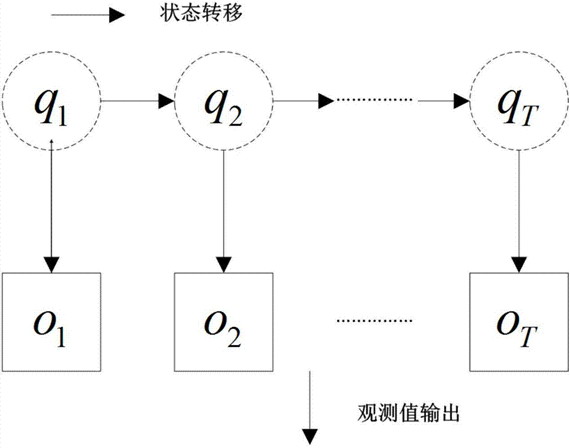在生成观测序列时我们需要考虑全局最优策略，而且我们更多的时候关心的不是生成的观测而是生成观测的最佳状态序列，所以隐马尔可夫模型有三个基本问题：（1）评估问题；（2）学习问题；（3）解码问题。

2.3.1 评估问题

评估问题是给定模型μ=(A, B, π)和观测序列，计算在模型μ下观测序列O出现的概率P(O | μ)。评估问题是学习问题和解码问题的基础，它为学习问题和解码问题提供了基本要素。先看下面的示意图：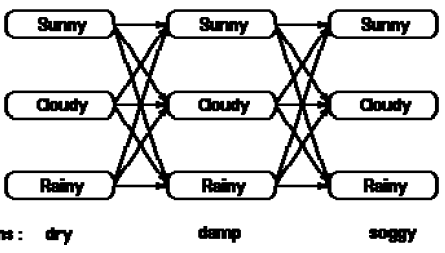最直接计算概率P(O | μ)的方式是计算每个时刻可能状态的转移概率和生成观测的概率并将所有可能路径的概率相加得到概率P(O | μ)。但是显而易见这种暴力计算的方式计算代价极为高昂，一个包含N个可能状态的HMM模型生成一个长度为T的状态序列的计算复杂度为

（1）前向概率

给定隐马尔科夫模型μ，定义到时刻t部分观测序列为且状态为的概率为前向概率，记作

前向概率的计算方式如下图所示：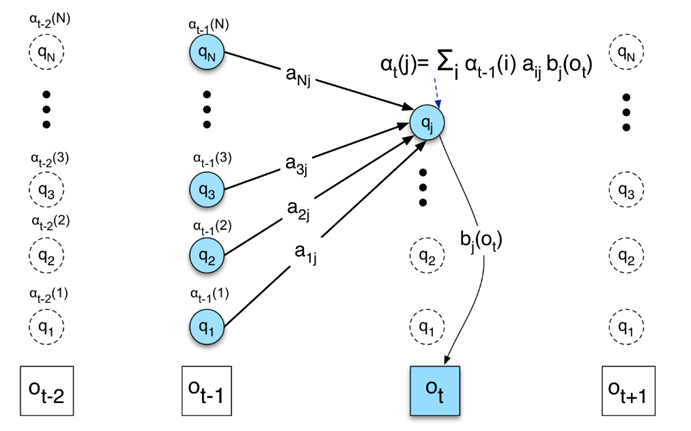t=1时刻状态i的前向概率为 ，t时刻状态i的前向概率为t-1时刻所有状态的前向概率转移到t时刻状态i的概率之和：

于是可得概率

（2）后向概率

给定隐马尔科夫模型μ，定义在时刻t状态为的条件下，从t+1到T的部分观测序列为的概率为后向概率，记作

初始化后向概率时，模型规定T时刻的，与前向概率相似，t时刻状态i的后向概率的递推公式为：

递推公式如下图所示：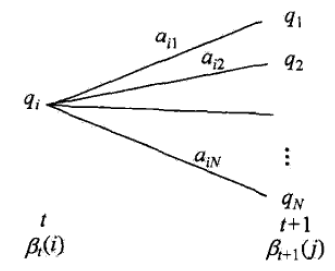最终概率

利用前向概率和后向概率的定义可以将观测序列概率P(O|μ)统一写成：

前向概率和后向概率运用动态规划算法降低了概率计算的复杂度，从暴力计算的降低到了，当T很大时，这个差别将非常大。

2.3.2 学习问题

已知观测序列，学习模型μ=(A, B, π)的参数，使得该模型下观测序列概率P(O | μ)最大。学习问题解决的是隐马尔科夫模型的训练问题，通过训练数据得到模型的参数A，B，π。根据训练数据是否包含观测序列相应的状态序列，学习问题可以用监督学习和无监督学习的方式训练。

监督学习的方式，是给定训练数据包含S个长度相同的观测序列和对应的状态序列，利用极大似然估计来估计隐马尔科夫模型的参数：

（1）转移概率的估计

设样本中时刻t处于状态i时刻t+1转移到状态j的频数为，那么状态转移概率的估计是：

（2）观测概率的估计

设样本中状态为j并观测为k的频数，那么状态为j观测为k的概率的估计是：

（3）初始状态概率πi的估计为S个样本中初始状态为的频率。

当 给定训练数据只包含S个长度为T的观测序列而没有对应的状态序列时，那么隐马尔科夫模型是一个以观测序列数据为观测数据O，以状态序列数据为不可观测隐数据I的概率模型：

此时参数的学习可以使用Baum-Welch算法实现，这是一种期望最大化算法（EM算法）。Baum-Welch算法的输入输如下：

输入：观测数据

输出：隐马尔科夫模型参数 μ = (A, B, π)

则算法步骤如下：

（1）初始化

对 n=0，选取，得到模型

（2）递推。对n=1，2，……，

上面几式等号右边以观测和模型计算。式中

其中是前向概率，是后向概率。

（3）终止。得到模型参数

Baum-Welch算法也叫前向-后向算法，因为其中的参数计算用到了前向概率和后向概率。第二步递推公式在李航博士的《统计学习方法论》的隐马尔可夫模型一章给出了详细的推导，有兴趣的读者可以仔细阅读。

2.3.3 解码问题：

已知模型μ=(A, B, π)和观测序列，求对给定观测序列条件概率P(I | O)最大的状态序列，即给定观测序列，求最有可能的对应的状态序列。在参数学习完成之后，解码问题是隐马尔科夫模型模型实际应用过程中的预测问题。比如在词性标注中给定由单词组成的句子（观测序列），输出句子单词相应词性的序列（状态序列）。

解决解码问题最直觉的方式，是在每个时刻t选择在该时刻最优可能出现的状态，从而得到一个状态序列，将它作为预测的结果。例如，给定隐马尔科夫模型μ和观测序列O，在时刻t处于状态的概率 γ 如上文的等式所定义，在每一时刻t最有可能的状态是：

从而得到状态序列。这种直接计算的近似算法，计算简单，但是一种典型的贪心算法，没有考虑全局最优，所以缺点是不能保证预测的状态序列整体是最有可能的状态序列。

解决解码问题最经典的算法是维特比算法。维特比算法也是动态规划的典型应用。它基于最优路径的这样一个特性：如果最优路径在时刻t通过节点，那么这一路径从节点到终点的部分路径，对于从的所有可能的部分路径来说，必须是最优的。（至于原因，非常简单，读者可以自行思考一下）

在说明维特比算法之前，我们首先定义维特比变量，为在t时刻状态为i的所有单个路径中概率最大值：

维特比变量的递推公式为：

细心的读者可能已经发现了，维特比变量的递推公式和前向概率（变量）的递推公式十分相似，实际上，前向变量是t时刻状态为i的所有路径的概率之和，维特比变量是t时刻状态为i的单个路径的概率最大值，如下图所示：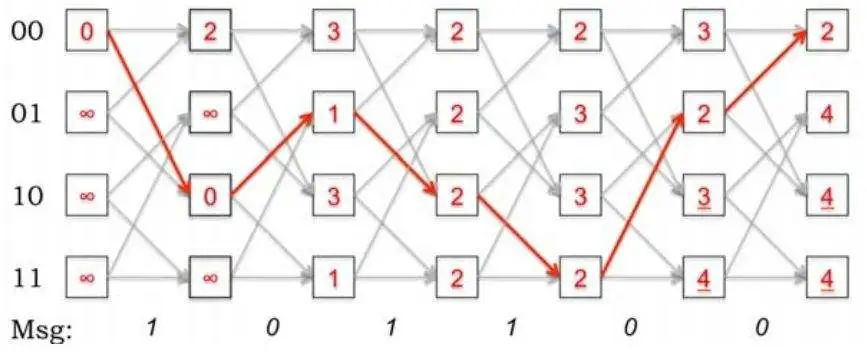由上图可以看出（实际上上图不是隐马尔科夫模型，但是比较契合维特比变量和前向概率的直观比较，我实在找不到合适的图了），红色的路径代表维特比变量的路径，前向概率的路径是所以路径的加和。除了维特比变量，维特比算法定义了在时刻t状态为i的所有单个路径中概率最大的路径的第t-1个节点的变量：

于是维特比算法流程如下：

输入：模型 μ=(A, B, π) 和观测

输出：最优路径

（1）初始化

（2）递推。对t = 2, 3, …… , T

（3）终止

（4）最优路径回溯。对t = T-1, T-2, ……, 1

求得最优路径

3. 总结

以上就是马尔可夫模型的基本内容，作为一种简单的概率图模型，隐马尔可夫模型有着广泛的应用，例如概率上下文文法可以认为是隐马尔科夫模型模型的一种推广，动态贝叶斯网络（dynamic Bayesian network）包含了隐马尔科夫模型。虽然隐马尔可夫模型也有着一些缺点，比如过强的独立性假设以及标记偏置问题，使得隐马尔可夫模型不像条件随机场那么强大。

同时，在具体的实现过程中，前向概率等应用了动态规划，可以用递归算法实现，但是递归实现的简单性往往会掩盖算法本身的复杂性，当观测序列很长时，尤其是隐马尔科夫模型训练用的是观测序列的总长度，用递归算法往往可能会出现栈溢出的情况，尤其像python这种没有尾递归优化的语言，在实现的时候必须考虑这个问题。总之，隐马尔科夫模型模型是一个相对简单的序列标注模型，有兴趣的读者可以自己实现一下。

展开全文机器学习
• 资源非常优秀, 且为最新的课程资源, 详细解释马尔可夫模型的由来和推导, 并伴随着丰富的实例, 让人很容易理解这门课程,
• 论文研究-基于隐马尔科夫模型的中国股票信息探测.pdf, 应用隐马尔科夫模型对不可观测的股票信息状态建模, 并构建信息状态转移概率矩阵刻画信息状态在时间维度上的动态...
• 隐式马尔科夫MATLAB代码小集合，可用于预测拟合等研究
• 资源名：隐马尔科夫模型_matlab 资源类型：matlab项目全套源码 源码说明： 全部项目源码都是经过测试校正后百分百成功运行的，如果您下载后不能运行可联系我进行指导或者更换。 适合人群：新手及有一定经验的开发...
• 文章目录一、马尔科夫链二、隐马尔科夫模型原理三个基本问题三、概率计算1. 前向算法2. 后向算法3. 常用的概率计算三、实际应用四、模型训练(学习)1. 监督学习2. Baum-Welch算法五、模型预测维特比(Viterbi)算法 ...

### 文章目录

隐马尔科夫模型（Hidden Markov Model，HHM）是一种有向图模型，属于生成式模型，考虑联合概率分布。

HHM有两组变量，第一组是状态变量 I = { i 1 , i 2 , . . . . . , i T } I = \{i_1, i_2,....., i_T\} i t i_t 表示第t时刻的状态，通常假定状态变量是不可被观测的、隐藏的，这应该也是名字的由来吧；

第二组变量是观测变量， O = { o 1 , o 2 , . . . . , o T } O = \{o_1, o_2,....,o_T\} o t o_t 表示第t时刻的观测值。

为了更好地理解，我们这是假设O是离散变量，并且假定O的取值范围为 V = v 1 , v 2 , . . . , v M V={v_1, v_2,...,v_M}

还有一个状态I的枚举值，即不同时刻的状态是在 Q = { q 1 , q 2 , . . . . . , q N } Q=\{q_1, q_2,.....,q_N\} 之间转换的。

# 一、马尔科夫链

首先，在了解HHM之前，我们需要知道**马尔科夫链：**在任一时刻t，观测变量的取值 o t o_t 仅依赖于当前的状态变量 i t i_t ，与其他时刻的状态变量及观测变量无关；同时，当前的状态值 i t i_t 仅依赖于前一个时刻的状态 i t − 1 i_{t-1} ，与状态无关。

如下图：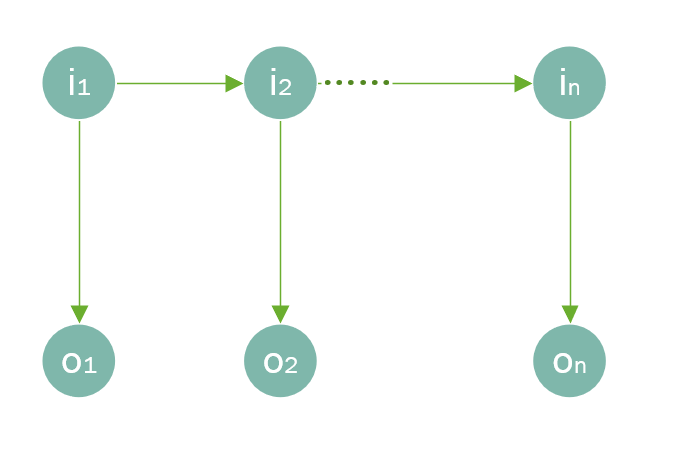# 二、隐马尔科夫模型原理

HHM就是基于这种马尔科夫链的，即基于以上的假设，所以它的联合概率分布为：

P ( o 1 , i 1 , . . . , o T , i T ) = P ( i 1 ) P ( o 1 ∣ i 1 ) ∏ i = 2 T P ( i i ∣ i i − 1 ) P ( o i ∣ i i ) P(o_1,i_1,...,o_T,i_T) = P(i_1)P(o_1|i_1)\prod_{i=2}^T P(i_i|i_{i-1})P(o_i|i_i)

这就是HHM的模型结构了。一个模型肯定还包含参数，机器学习的本质就是找到一组最优的参数，使得模型的拟合效果最好，HHM也不例外。

那么，HHM的参数包括以下3组：

1. 状态转移概率A： a i j = P ( i t + 1 = q j ∣ i t = q i ) , 1 < = i , j < = N a_{ij} = P(i_{t+1}=q_j|i_t=q_i), 1<=i,j<=N ，即t时刻状态为 q i q_i 时，t+1时刻状态为 q j q_j 的概率
2. 输出观测概率B： b j ( k ) = P ( o t = q k ∣ i t = q j ) , 1 < = j < = N , 1 < = k < = M b_j(k) = P(o_t=q_k|i_t=q_j), 1<=j<=N,1<=k<=M ，即t时刻的状态为 q i q_i 时，观测值为 o j o_j 的概率
3. 初设状态概率 π i \pi_i π = ( π 1 , π 2 , . . . , π N ) , 1 < = i < = N \pi = (\pi_1, \pi_2,...,\pi_N), 1<=i<=N ，即初设时刻各状态出现的概率。

那么，给定一个隐马尔科夫模型，它产生观测序列的步骤如下 { o 1 , o 2 , . . . . , o T } \{o_1, o_2,....,o_T\}

(1) 当t = 1时，根据初设状态概率 π \pi 选择初设状态 i 1 i_1

(2) 根据状态 i t i_t 和输出观测概率B选择观测值 o t o_t

(3) 根据状态 i t i_t 和状态转移概率A确定 i t + 1 i_{t+1}

(4) 当t < T，令t = t + 1，并跳转至第2步，否则终止，观测序列已全部生产完毕。

## 三个基本问题

1. 概率计算问题。给定模型 λ = ( A , B , π ) \lambda=(A,B,\pi) 和观测序列 O = { o 1 , o 2 , . . . . , o T } O = \{o_1, o_2,....,o_T\} ，计算观测序列O出现的概率 P ( O ∣ λ ) P(O|\lambda)
2. 学习问题。已知观测序列 O = { o 1 , o 2 , . . . . , o T } O = \{o_1, o_2,....,o_T\} ，估计模型参数 λ = ( A , B , π ) \lambda=(A,B,\pi) ，使得观测序列概率 P ( O ∣ λ ) P(O|\lambda) 最大
3. 预测问题。给定模型 λ = ( A , B , π ) \lambda=(A,B,\pi) 和观测序列 O = { o 1 , o 2 , . . . . , o T } O = \{o_1, o_2,....,o_T\} ，求对给定观测序列条件概率P(I|O)最大的隐藏状态 I = { i 1 , i 2 , . . . . . , i T } I = \{i_1, i_2,....., i_T\}

# 三、概率计算

## 1. 前向算法

给定隐马尔科夫模型 λ \lambda ，至时刻t的观测序列为 { o 1 , o 2 , . . . . , o t } \{o_1, o_2,....,o_t\} ，且状态为 q i q_i 的概率为前向概率：

α t ( i ) = P ( o 1 , o 2 , . . . . , o t , i t = q i ∣ λ ) \alpha_t(i)=P(o_1, o_2,....,o_t,i_t=q_i|\lambda)

我们可以通过递推的方式求得前向概率 α t ( i ) \alpha_t(i) 及观测序列概率 P ( O ∣ λ ) P(O|\lambda) ，具体的算法步骤如下：

(1) 时刻t=1， α 1 ( i ) = π i b i ( o 1 ) , i = 1 , 2 , . . . , N \alpha_1(i)=\pi_ib_i(o_1),i=1,2,...,N

(2) 对于时刻 t = 1 , 2 , . . . , T − 1 t=1,2,...,T-1

α t + 1 ( i ) = [ ∑ j = 1 N α t ( j ) a j i ] b i ( o t + 1 ) , i = 1 , 2 , . . . , N \alpha_{t+1}(i)=\left[\sum_{j=1}^N\alpha_t(j)a_{ji}\right]b_i(o_{t+1}),i=1,2,...,N

(3) P ( O ∣ λ ) = ∑ i = 1 N α T ( i ) P(O|\lambda)=\sum^N_{i=1}\alpha_T(i)

## 2. 后向算法

给定隐马尔科夫模型 λ \lambda ，满足时刻t的状态为 q i q_i 的条件下，从时刻t+1到T的观测序列为 { o t + 1 , o t + 2 , . . . . , o T } \{o_{t+1}, o_{t+2},....,o_T\} 的概率为后向概率：

β t ( i ) = P ( o t + 1 , o t + 2 , . . . . , o T ∣ i t = q i , λ ) \beta_t(i)=P(o_{t+1}, o_{t+2},....,o_T|i_t=q_i,\lambda)

我们同样可以通过递推的方式求得前向概率 β t ( i ) \beta_t(i) 及观测序列概率 P ( O ∣ λ ) P(O|\lambda) ，具体的算法步骤如下：

(1) 对于最后时刻T，规定 β T ( i ) = 1 \beta_T(i)=1

(2) 对于时刻t=T-1, T-2, … , 1：

β t ( i ) = ∑ j = 1 N a i j b j ( o t + 1 ) β t + 1 ( j ) , i = 1 , 2 , . . . , N \beta_t(i)=\sum^N_{j=1}a_{ij}b_j(o_{t+1})\beta_{t+1}(j), i=1,2,...,N

(3) P ( O ∣ λ ) = ∑ i = 1 N π i b i ( o 1 ) β 1 ( i ) P(O|\lambda)=\sum^N_{i=1}\pi_ib_i(o_1)\beta_1(i)

这里我们可以这里理解 ： β 1 ( i ) \beta_1(i) 表示时刻t=1的状态为 q 1 q_1 时，从时刻t=2到T的观测序列为 { o 2 , o 3 , . . . . , o T } \{o_{2}, o_{3},....,o_T\} 的概率， π i b i ( o 1 ) \pi_ib_i(o_1) 当然就是时刻t=1的状态为 q 1 q_1 的概率了，那么 π i b i ( o 1 ) β 1 ( i ) \pi_ib_i(o_1)\beta_1(i) 也就是时刻t=1的状态为 q 1 q_1 的条件下的条件概率了。

但是观测序列概率是没有限定观测序列的状态取值的，所以，要把所有状态下的观测序列概率累加起来才是最后的观测序列概率，即上式。

这里跟上面的前向算法求得的观测序列概率的思想是类似的。

## 3. 常用的概率计算

1. 根据已知的隐马尔科夫模型 λ \lambda 和观测序列O，在时刻 t 处于状态 q i q_i 的概率：

γ t ( i ) = P ( i t = q i ∣ O , λ ) \gamma_t(i)=P(i_t=q_i|O,\lambda)

并且，根据条件概率的公式进行推导可知：

γ t ( i ) = P ( i t = q i ∣ O , λ ) = P ( i t = q i , O ∣ λ ) P ( O ∣ λ ) \gamma_t(i)=P(i_t=q_i|O,\lambda)=\frac{P(i_t=q_i,O|\lambda)}{P(O|\lambda)}

再结合前向和后向概率，可得到：

P ( i t = q i , O ∣ λ ) = α t ( i ) β t ( i ) P(i_t=q_i,O|\lambda)=\alpha_t(i)\beta_t(i)

（这里多琢磨几遍前向概率和后向概率的定义，就可以理解为什么了）

则：

γ t ( i ) = α t ( i ) β t ( i ) P ( O ∣ λ ) = α t ( i ) β t ( i ) ∑ j = 1 N α t ( j ) β t ( j ) \gamma_t(i)=\frac{\alpha_t(i)\beta_t(i)}{P(O|\lambda)}=\frac{\alpha_t(i)\beta_t(i)}{\sum^N_{j=1}\alpha_t(j)\beta_t(j)}

（分母的转换是这么理解：在已知 λ \lambda 的情况下，观测序列为O时，状态可以为任何一种状态，那么每一种状态下观测序列为O的概率累加，就是观测序列的概率了）

2. 根据已知的隐马尔科夫模型 λ \lambda 和观测序列O，在时刻 t 处于状态 q i q_i ，并且在时刻t+1处于状态 q j q_j 的概率：

ξ t ( i , j ) = P ( i t = q i , i t + 1 = q j ∣ O , λ ) \xi_t(i,j)=P(i_t=q_i,i_{t+1}=q_j|O,\lambda)

跟上面的思路一致：

ξ t ( i , j ) = P ( i t = q i , i t + 1 = q j , O ∣ λ ) P ( O ∣ λ ) = P ( i t = q i , i t + 1 = q j , O ∣ λ ) ∑ i = 1 N ∑ j = 1 N P ( i t = q i , i t + 1 = q j , O ∣ λ ) \xi_t(i,j)=\frac{P(i_t=q_i,i_{t+1}=q_j,O|\lambda)}{P(O|\lambda)}=\frac{P(i_t=q_i,i_{t+1}=q_j,O|\lambda)}{\sum^N_{i=1}\sum^N_{j=1}P(i_t=q_i,i_{t+1}=q_j,O|\lambda)}

P ( i t = q i , i t + 1 = q j , O ∣ λ ) = α t ( i ) a i j b j ( o t + 1 ) β t + 1 ( j ) P(i_t=q_i,i_{t+1}=q_j,O|\lambda)=\alpha_t(i)a_{ij}b_j(o_{t+1})\beta_{t+1}(j)

# 三、实际应用

可能看了上面隐马尔科夫模型的原理之后，很多人都有点懵，不知道能用来做什么？

我们举个例子：中文分词。

“我们是中国人”，这一句话分词之后应该是这样的：我(B)们(E)|是(O)|中(B)国(M)人(E)。

各个标签的含义——B：单词的第一个字，M：单词的中间字，E：单词的最后一个字，O：仅有一个字组成的词。

将隐马尔科夫模型应用到分词中，那么观测序列对应的是文本句子，隐藏状态对应则的是句子中每个字的标签。

# 四、模型训练(学习)

上面我们提到了，HHM的参数有三组 A , B , π {A,B,\pi} ，那么如何确定最优的参数呢？

## 1. 监督学习

当我们的样本数据是带有标签时，即训练数据包含观测序列以及对应的状态，我们就可以通过极大似然法来估计HHM的参数，计算方法如下：

(1) 状态转移概率A: a ^ i j = A i j ∑ k = 1 N A i k , 1 < = i , j < = N \hat{a}_{ij} = \frac{A_{ij}}{\sum_{k=1}^NA_{ik}},1<=i,j<=N

A i j A_{ij} 表示样本中时刻t处于状态 q i q_i 转移到时刻t+1处于状态 q j q_j 的频次。

如上面分词的例子， A i j A_{ij} 可以理解为当前这个字的标签为 q i q_i (假如为B)，下一个词的标签为 q j q_j （假如为E）的频次。

(2) 输出观测概率B： b ^ i j = B i j ∑ k = 1 N B i k , 1 < = i < = N , 1 < = j < = M \hat{b}_{ij} = \frac{B_{ij}}{\sum_{k=1}^NB_{ik}},1<=i<=N,1<=j<=M

B i j B_{ij} 表示样本中t时刻的状态为 q i q_i 时，观测值为 o j o_j 的频次。

如当前的字为 o j o_j （假如为"我"），标签为 q i q_i （假如为B）的频次。

(3) 初设状态概率 π \pi π ^ i \hat{\pi}_i 为初设状态为 q i q_i 的频率。

## 2. Baum-Welch算法

如果样本数据时没有带标签的，即训练数据只包含观测序列O，但对应的状态 I I 未知。

那此时的隐马尔科夫模型是一个含有隐变量的概率模型：

P ( O ∣ λ ) = ∑ I P ( O ∣ I , λ ) P ( I ∣ λ ) P(O|\lambda)=\sum_IP(O|I,\lambda)P(I|\lambda)

它的参数学习可以由Baum-Welch算法实现，本质还是EM。EM的基本思想是先将参数的初设估计值加入到似然函数Q中，然后对Q进行极大化（一般就是求导，令其等于0），得到新的参数估计值，一直重复，直到收敛。

此时，对数似然函数是 l o g P ( O , I ∣ λ ) logP(O,I|\lambda)

(1) EM算法的E步：求Q函数：

Q ( λ , λ ˉ ) = ∑ I l o g P ( O , I ∣ λ ) P ( O , I ∣ λ ˉ ) Q(\lambda,\bar{\lambda})=\sum_IlogP(O,I|\lambda)P(O,I|\bar{\lambda})

λ \lambda 是要极大化的隐马尔科夫模型的参数， λ ˉ \bar{\lambda} 是参数的当前估计值。

因为 P ( O , I ∣ λ ) = π i 1 b i 1 ( o 1 ) a i 1 i 2 b i 2 . . . a i T − 1 i T b i T ( o T ) P(O,I|\lambda)=\pi_{i1}b_{i_1}(o_1)a_{i_1i_2}b_{i_2}...a_{i_{T-1}i_T}b_{i_T}(o_T)

因此 Q ( λ , λ ˉ ) = ∑ I l o g π i 1 P ( O , I ∣ λ ˉ ) + ∑ I ( ∑ t = 1 T − 1 l o g α i t i t + 1 ) P ( O , I ∣ λ ˉ ) + ∑ I ( ∑ t = 1 T l o g b i t ( o t ) ) P ( O , I ∣ λ ˉ ) Q(\lambda,\bar{\lambda})=\sum_Ilog\pi_{i_1}P(O,I|\bar{\lambda})+\sum_I\left(\sum^{T-1}_{t=1}log\alpha_{i_ti_{t+1}}\right)P(O,I|\bar{\lambda})+\sum_I\left(\sum^T_{t=1}logb_{i_t}(o_t)\right)P(O,I|\bar{\lambda})

(2) EM算法的M步：极大化Q函数，得到新的参数估计值 A , B , λ A,B,\lambda

由于三个参数单独出现在Q函数的3个项中，那么我们可以对各项分别极大化。

a. 首先是第1项求 λ \lambda

∑ I l o g π i 1 P ( O , I ∣ λ ˉ ) = ∑ i = 1 N l o g π i P ( O , i 1 = i ∣ λ ˉ ) \sum_Ilog\pi_{i_1}P(O,I|\bar{\lambda})=\sum^N_{i=1}log\pi_iP(O,i_1=i|\bar{\lambda})

为什么这个等式成立呢？因为当隐马尔科夫模型的参数确定后，时刻t=1的状态 i 1 i_1 确定了，那么O和 I I 都能确定，这里是把 i 1 i_1 的所有独立事件概率加起来

π i \pi_i 满足约束条件 ∑ i = 1 N π = 1 \sum^N_{i=1}\pi=1 ，可以利用拉格朗日乘子法，拉格朗日函数为：

∑ i = 1 N l o g π i P ( O , i 1 = i ∣ λ ˉ ) + γ ( ∑ i = 1 N π i − 1 ) \sum^N_{i=1}log\pi_iP(O,i_1=i|\bar{\lambda})+\gamma\left(\sum^N_{i=1}\pi_i-1\right)

求偏导并令其等于0：

∂ ∂ π i [ ∑ i = 1 N l o g π i P ( O , i 1 = i ∣ λ ˉ ) + γ ( ∑ i = 1 N π i − 1 ) ] = 0 \frac{\partial}{\partial \pi_i}\left[\sum^N_{i=1}log\pi_iP(O,i_1=i|\bar{\lambda})+\gamma\left(\sum^N_{i=1}\pi_i-1\right)\right]=0

得到： P ( O , i 1 = i ∣ λ ˉ ) + γ π i = 0 P(O,i_1=i|\bar{\lambda})+\gamma\pi_i=0

对所有i求和，得到： γ = − P ( O ∣ λ ˉ ) \gamma=-P(O|\bar{\lambda})

因为 i 1 ∈ ( 1 , 2 , . . . , N ) i_1\in(1,2,...,N) ，所以 P ( O , i 1 = i ∣ λ ˉ ) P(O,i_1=i|\bar{\lambda}) 中按 i i 从1到N累计起来其实就等于 P ( O ∣ λ ˉ ) P(O|\bar{\lambda})

最终， π i = P ( O , i 1 = i ∣ λ ˉ ) P ( O ∣ λ ˉ ) \pi_i=\frac{P(O,i_1=i|\bar{\lambda})}{P(O|\bar{\lambda})}

b. Q函数的第2项求a：

∑ I ( ∑ t = 1 T − 1 l o g α i t i t + 1 ) P ( O , I ∣ λ ˉ ) = ∑ i = 1 N ∑ j = 1 N ∑ t = 1 T − 1 a i j P ( O , i t = i , i t + 1 = j ∣ λ ˉ ) \sum_I\left(\sum^{T-1}_{t=1}log\alpha_{i_ti_{t+1}}\right)P(O,I|\bar{\lambda})=\sum^N_{i=1}\sum^N_{j=1}\sum^{T-1}_{t=1}a_{ij}P(O,i_t=i,i_{t+1}=j|\bar{\lambda})

同样存在约束条件： ∑ j = 1 N a i j = 1 \sum^N_{j=1}a_{ij}=1 ，类似上面的做法，可以求得：

a i j = ∑ t = 1 T − 1 P ( O , i t = i , i t + 1 = j ∣ λ ˉ ) ∑ t = 1 T − 1 P ( O , i t = i ) ∣ λ ˉ a_{ij}=\frac{\sum^{T-1}_{t=1}P(O,i_t=i,i_{t+1}=j|\bar{\lambda})}{\sum^{T-1}_{t=1}P(O,i_t=i)|\bar{\lambda}}

c. Q函数的第3项求b：

∑ I ( ∑ t = 1 T l o g b i t ( o t ) ) P ( O , I ∣ λ ˉ ) = ∑ j = 1 N ∑ t = 1 T l o g b j ( o t ) i P ( O , i t = j ∣ λ ˉ ) \sum_I\left(\sum^T_{t=1}logb_{i_t}(o_t)\right)P(O,I|\bar{\lambda})=\sum^N_{j=1}\sum^T_{t=1}logb_j(o_t)_iP(O,i_t=j|\bar{\lambda})

约束条件： ∑ k = 1 M b j ( k ) = 1 \sum^M_{k=1}b_j(k)=1 ，这里需要注意只有当 o t = v k o_t=v_k b j ( o t ) b_j(o_t) b j ( k ) b_j(k) 的偏导数才不等于0，用 I ( o t = v k ) I(o_t=v_k) 表示，求得：

b j ( k ) = ∑ t = 1 T P ( O , i t = j ∣ λ ˉ ) I ( o t = v k ) ∑ t = 1 T P ( O , i t = j ∣ λ ˉ ) b_j(k)=\frac{\sum^T_{t=1}P(O,i_t=j|\bar{\lambda})I(o_t=v_k)}{\sum^T_{t=1}P(O,i_t=j|\bar{\lambda})}

(3) 最后，用上面的概率表示：

a i j = ∑ t = 1 T − 1 ξ t ( i , j ) ∑ t = 1 T − 1 γ t ( i ) a_{ij}=\frac{\sum^{T-1}_{t=1}\xi_t(i,j)}{\sum^{T-1}_{t=1}\gamma_t(i)}

b j ( k ) = ∑ t = 1 , o t = v k T γ t ( j ) ∑ t = 1 T γ t ( j ) b_j(k)=\frac{\sum^T_{t=1,o_t=v_k}\gamma_t(j)}{\sum^T_{t=1}\gamma_t(j)}

π i = γ 1 ( i ) \pi_i=\gamma_1(i)

# 五、模型预测

那么，通过训练，我们得到了最优的参数，也相当于我们得到了一个拟合效果最好的隐马尔科夫模型，此时我们如何预测观测序列的状态呢？即给定隐马尔科夫模型 λ = [ A , B , π ] \lambda=[A,B,\pi] ，如何通过观测序列来推断对应的隐藏状态。

举个例子：例如我们这个模型用于语音识别中，那么观测序列就是语音了，隐藏状态即为文字。语音识别的作用就是将语音转化为对应的文字，那如果隐马尔科夫模型通过观测序列得到隐藏状态，不就是将语音转化为文字了。

这个时候就需要使用到维特比(Viterbi)算法，在这篇博客中有详细介绍。但在隐马尔科夫模型中需要稍作调整，下面我给出书上的一个例子。

## 维特比(Viterbi)算法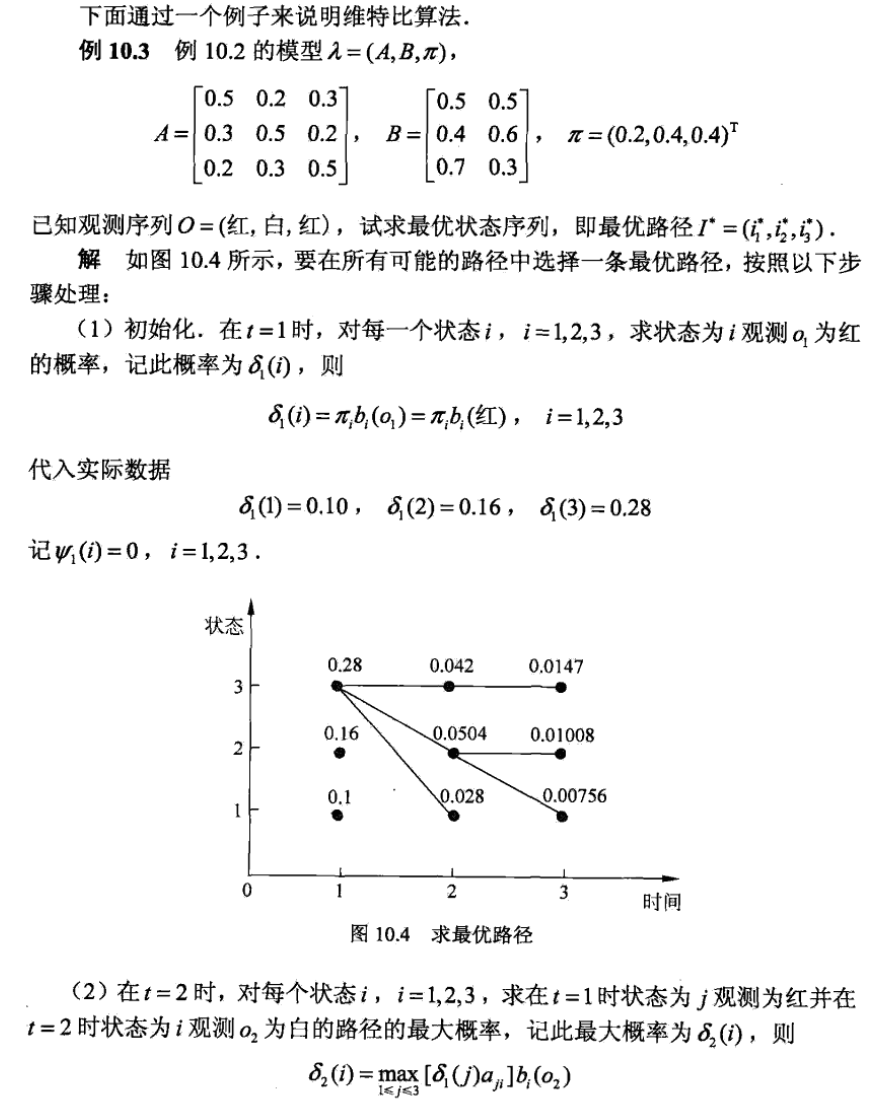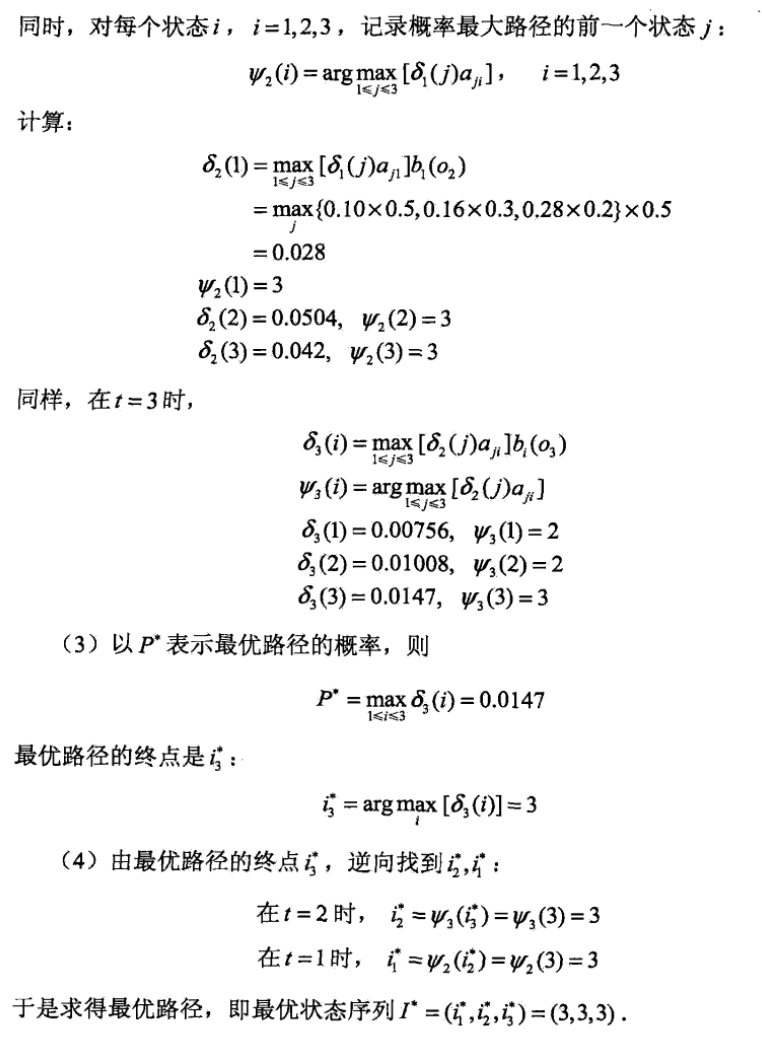欢迎关注同名公众号：“我就算饿死也不做程序员”。
交个朋友，一起交流，一起学习，一起进步。展开全文HMM 自然语言处理 马尔科夫
• 使用隐马尔科夫模型（Hidden Markov Model，HMM） 进行分词，并与基于词典的正向最大匹配算法和工业界使用的jieba分词进行对比。 采用最大似然估计的方法从带标记样本学习模型参数，并通过维特比算法进行解码。
• 文章目录什么是隐马尔科夫模型HMM模型相关的算法前向算法(forward algorithm)维特比算法(Viterbi algorithm)求解最可能的天气NLP应用小结 参考文章一文搞懂HMM（隐马尔可夫模型） 本人博客地址: ...

### 文章目录

参考文章 一文搞懂HMM（隐马尔可夫模型）
本人博客地址: https://xiaoxiablogs.top

## 什么是隐马尔科夫模型

隐马尔可夫模型（Hidden Markov Model，HMM）是统计模型，它用来描述一个含有隐含未知参数的马尔可夫过程。其难点是从可观察的参数中确定该过程的隐含参数。然后利用这些参数来作进一步的分析，例如模式识别。用一个简单的例子来描述:

假设有3个不同的骰子，分别为四面(D4)、六面(D6)、八面(D8)，每个面的概率都是相同的，每个骰子被选中的概率也是相同的。

假设我们开始掷骰子，随机从三个里面选一个，然后掷骰子，我们会得到一个数字，重复上面的过程，我们就可以得到一串数字。例如我们得到的是1 8 6 4 4 2 3 6 1 4，这串数字就是可见状态链，而我们对应的掷骰子的顺序就是隐含状态链，假设为D4 D8 D6 D4 D6 D8 D6 D6 D4 D8，我们第一次随机的骰子是D4, 而第一次选中每个骰子的概率是1/3，也就是初始概率， 而第一次选中D4后，第二次选中每个骰子的概率为1/3，这就是转换概率，如:D4->D8为1/3，即选中D4后再选中D8的转移概率为1/3。

一般来说，HMM中说到的马尔科夫链其实就是指隐含状态链，因为隐含状态之间存在转换概率

## HMM模型相关的算法

1. 知道隐含状态数量、转换概率、可见状态链、输出概率，求隐含状态链
2. 知道隐含状态数量、转换概率、可见状态链、输出概率，求这个结果的概率
3. 知道隐含状态数量、隐含状态链、可见状态链、输出概率，求转换概率

## 前向算法(forward algorithm)

我们拿到一串可见状态链，也知道转换概率、输出概率，如何求解隐含状态链最可能是什么呢?

我们就可以使用前向算法(forward algorithm)

例如我们得到的数字为: 1 6 3

我们可以分为以下几步:

首先，我们只掷一次骰子:看到结果为1，产生这个结果的总概率可以按照如下计算，清高率为0.18：然后我们掷两次骰子:看到结果为1，6.产生这个结果的总概率可以按照如下计算，总概率为0.05：然后我们掷三次骰子:看到结果为1，6，3.产生这个结果的总概率可以按照如下计算，总概率为0.03：同样的，我们一步一步的算，有多长算多长，再长的马尔可夫链总能算出来的。这就是前向算法(forward algorithm)

## 维特比算法(Viterbi algorithm)

任何一个HMM都可以通过下列五元组来描述：

:param obs:观测序列
:param states:隐状态
:param start_p:初始概率（隐状态）
:param trans_p:转移概率（隐状态）
:param emit_p: 发射概率 （隐状态表现为显状态的概率）states = ('Rainy', 'Sunny')

observations = ('walk', 'shop', 'clean')

start_probability = {'Rainy': 0.6, 'Sunny': 0.4}

transition_probability = {
'Rainy' : {'Rainy': 0.7, 'Sunny': 0.3},
'Sunny' : {'Rainy': 0.4, 'Sunny': 0.6},
}

emission_probability = {
'Rainy' : {'walk': 0.1, 'shop': 0.4, 'clean': 0.5},
'Sunny' : {'walk': 0.6, 'shop': 0.3, 'clean': 0.1},
}


### 求解最可能的天气

求解最可能的隐状态序列是HMM的三个典型问题之一，通常用维特比算法解决。维特比算法就是求解HMM上的最短路径（-log(prob)，也即是最大概率）的算法。

稍微用中文讲讲思路，很明显，第一天天晴还是下雨可以算出来：

1. 定义V[时间][今天天气] = 概率，注意今天天气指的是，前几天的天气都确定下来了（概率最大）今天天气是X的概率，这里的概率就是一个累乘的概率了。
2. 因为第一天我的朋友去散步了，所以第一天下雨的概率V[第一天][下雨] = 初始概率[下雨] * 发射概率[下雨][散步] = 0.6 * 0.1 = 0.06，同理可得V[第一天][天晴] = 0.24 。从直觉上来看，因为第一天朋友出门了，她一般喜欢在天晴的时候散步，所以第一天天晴的概率比较大，数字与直觉统一了。
3. 从第二天开始，对于每种天气Y，都有前一天天气是X的概率 * X转移到Y的概率 * Y天气下朋友进行这天这种活动的概率。因为前一天天气X有两种可能，所以Y的概率有两个，选取其中较大一个作为V[第二天][天气Y]的概率，同时将今天的天气加入到结果序列中
4. 比较V[最后一天][下雨]和[最后一天][天晴]的概率，找出较大的哪一个对应的序列，就是最终结果。
# -*- coding:utf-8 -*-
# Filename: viterbi.py
# Author：hankcs
# Date: 2014-05-13 下午8:51

states = ('Rainy', 'Sunny')

observations = ('walk', 'shop', 'clean')

start_probability = {'Rainy': 0.6, 'Sunny': 0.4}

transition_probability = {
'Rainy' : {'Rainy': 0.7, 'Sunny': 0.3},
'Sunny' : {'Rainy': 0.4, 'Sunny': 0.6},
}

emission_probability = {
'Rainy' : {'walk': 0.1, 'shop': 0.4, 'clean': 0.5},
'Sunny' : {'walk': 0.6, 'shop': 0.3, 'clean': 0.1},
}

# 打印路径概率表
def print_dptable(V):
print "    ",
for i in range(len(V)): print "%7d" % i,
print

for y in V.keys():
print "%.5s: " % y,
for t in range(len(V)):
print "%.7s" % ("%f" % V[t][y]),
print

def viterbi(obs, states, start_p, trans_p, emit_p):
"""

:param obs:观测序列
:param states:隐状态
:param start_p:初始概率（隐状态）
:param trans_p:转移概率（隐状态）
:param emit_p: 发射概率 （隐状态表现为显状态的概率）
:return:
"""
# 路径概率表 V[时间][隐状态] = 概率
V = [{}]
# 一个中间变量，代表当前状态是哪个隐状态
path = {}

# 初始化初始状态 (t == 0)
for y in states:
V[y] = start_p[y] * emit_p[y][obs]
path[y] = [y]

# 对 t > 0 跑一遍维特比算法
for t in range(1, len(obs)):
V.append({})
newpath = {}

for y in states:
# 概率 隐状态 =    前状态是y0的概率 * y0转移到y的概率 * y表现为当前状态的概率
(prob, state) = max([(V[t - 1][y0] * trans_p[y0][y] * emit_p[y][obs[t]], y0) for y0 in states])
# 记录最大概率
V[t][y] = prob
# 记录路径
newpath[y] = path[state] + [y]

# 不需要保留旧路径
path = newpath

print_dptable(V)
(prob, state) = max([(V[len(obs) - 1][y], y) for y in states])
return (prob, path[state])

def example():
return viterbi(observations,
states,
start_probability,
transition_probability,
emission_probability)

print example()


输出:

           0       1       2
Rainy:  0.06000 0.03840 0.01344
Sunny:  0.24000 0.04320 0.00259
(0.01344, ['Sunny', 'Rainy', 'Rainy'])


## NLP应用

具体到分词系统，可以将天气当成“标签”，活动当成“字或词”。那么，几个NLP的问题就可以转化为：

• 词性标注：给定一个词的序列（也就是句子），找出最可能的词性序列（标签是词性）。如ansj分词和ICTCLAS分词等。
• 分词：给定一个字的序列，找出最可能的标签序列（断句符号：[词尾]或[非词尾]构成的序列）。结巴分词目前就是利用BMES标签来分词的，B（开头）,M（中间),E(结尾),S(独立成词）
• 命名实体识别：给定一个词的序列，找出最可能的标签序列（内外符号：[内]表示词属于命名实体，[外]表示不属于）。如ICTCLAS实现的人名识别、翻译人名识别、地名识别都是用同一个Tagger实现的。

## 小结

HMM是一个通用的方法，可以解决贴标签的一系列问题。

展开全文机器学习 自然语言处理
• 点击上方“小白学视觉”，选择加"星标"或“置顶”重磅干货，第一时间送达隐马尔科夫模型是（hidden Markov model，HMM）是可用于标注问题的统计学习模型，描述由隐藏的马尔科夫链随机生成观测序列的过程。...算法 python 机器学习 人工智能 java
• 利用隐马尔科夫模型识别黄瓜MAPK基因家族及其分子进化学分析，李微，张珍珠，促有丝分裂原活化蛋白激酶基因家族（mitogen-activated protein kinases gene family，MAPK gene family）级联在信号转导途径中起着重要...
• 隐马尔科夫模型 文章目录隐马尔科夫模型前言一、定义二、三个基本问题1、观测序列概率2、模型参数学习3、预测（解码）问题总结 前言 隐马尔科夫模型（HMM）是在马尔科夫链上的一个扩展，属于机器学习，它用来描述...matlab 机器学习
• 前言：前面的一片文章介绍了马尔科夫模型，以及里面的一些核心概念，如转移概率、状态、转移概率矩阵等，本次文章更进一步，介绍马尔可夫模型。它是在马尔科夫模型的基础之上进一步得来的。马尔可夫模型最重要的...HMM
• 资源名：whmt1_隐马尔科夫模型_matlab 资源类型：matlab项目全套源码 源码说明： 全部项目源码都是经过测试校正后百分百成功运行的，如果您下载后不能运行可联系我进行指导或者更换。 适合人群：新手及有一定经验的...
• 1.隐马尔科夫模型求解步骤（其它大部分模型也均遵循如下路径） 2. HMM定义 2.1 HMM的两个基本性质 2.2 HMM的确定​ 3.HMM的三个基本问题 3.1 概率计算问题 3.1.1直接计算（暴力算法） 3.1.2前向算法和后向...自然语言处理 机器学习
• 隐马尔科夫模型的定义 隐马尔科夫模型（hidden Markov model，HMM）描述由一个隐藏的马尔科夫链随机生成不可观测的状态随机序列，再由各个状态生成一个观测从而产生观测随机序列的过程。隐藏的马尔科夫链随机生成的...算法
• 马尔可夫模型是关于时序的概率模型，由一个隐藏的马尔科夫链随机生成不可观测的状态随机序列python hmm
• 运动人体检测和行为识别涉及...利用傅里叶-隐马尔科夫模型进行人体识别，能够有效提高人体行为识别率，本次测试单个行为的识别中平均识别率达到94%，要进行深入探究，进行复杂环境复杂动作的识别，促进相关工作的改进。
• 本发明涉及人工智能及模式识别技术领域，尤其是一种基于隐马尔科夫模型的动态手势切分识别方法。背景技术：随着手机触摸操作和人体跟踪识别的发展，人们体会到了手势交互方式具有以人为中心的自然性，简洁性，和直接...
• 隐马尔科夫模型是自然语言处理中很重要的一种算法。在此之前，我们首先给大家介绍马尔科夫链。马尔科夫链，因安德烈·马尔科夫得名，是指数学中具有马尔科夫性质的离散事件的随机过程。在给定当前知识或信息的情况下...深度学习 股票预测 HMM...